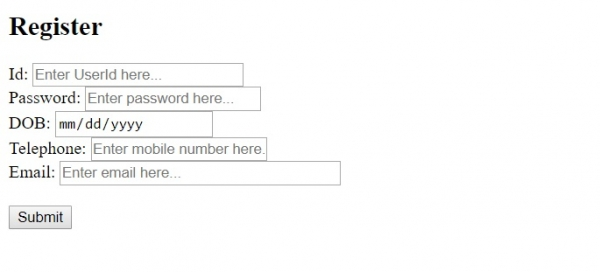# HTML <input> size Attribute

HTMLWeb DevelopmentFront End Technology

The size attribute of the <input> element is used to set the width of the input. The more the width would lead to a textbox with more width. You can set the size attribute for the following input types − text, search, email, password, tel and url.

Following is the syntax −

<input size="size_num">

Above, size_num is the width of the input you need to set in numbers. The default is 20.

Let us now see an example to implement the size attribute of the <input> element −

## Example

Live Demo

<!DOCTYPE html>
<html>
<body>
<h2>Register</h2>
<form action="" method="get">
Id − <input type="text" name="id" placeholder="Enter UserId here..." size = "25" required><br>
DOB − <input type="date" name="dob" placeholder="Enter date of birth here..."><br>
Telephone − <input type="tel" name="tel" placeholder="Enter mobile number here..." required><br>
Email − <input type="email" name="email" placeholder="Enter email here..." size = "35"><br><br>
<button type="submit" value="Submit">Submit</button>
</form>
</body>
</html>

## OutputIn the above example, we have some fields with a button −

<form action="" method="get">
Id − <input type="text" name="id" placeholder="Enter UserId here..." size = "25" required><br>
</form>
<input type="text" name="id" placeholder="Enter UserId here..." size = "25" required>